# Spectrum of a ring

A topological spacewhose points are the prime ideals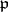of a ringwith the Zariski topology (also called the spectral topology). It is assumed thatis commutative and has an identity. The elements ofcan be regarded as functions on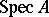by setting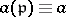.supports a sheaf of local rings, called its structure sheaf. For a point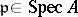, the stalk of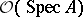overis the localization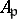ofat.
To any identity-preserving ring homomorphism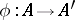there corresponds a continuous mapping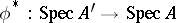. Ifis the nil radical of, then the natural mappingis a homeomorphism of topological spaces.
For a non-nilpotent element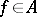, let, where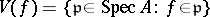. Then the ringed spacesand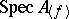, where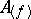is the localization ofwith respect to, are isomorphic. The sets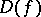are called the principal open sets. They form a basis for the topology on. A pointis closed if and only ifis a maximal ideal of. By assigning toits closure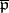in, one obtains a one-to-one correspondence between the points ofand the set of closed irreducible subsets of.is quasi-compact, but usually not Hausdorff. The dimension ofis defined as the largestfor which there is a sequence of distinct closed irreducible sets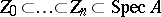.
Many properties ofcan be described in terms of. For example,is Noetherian if and only ifhas the descending chain condition for closed sets;is an irreducible space if and only if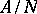is an integral domain; the dimension ofcoincides with the Krull dimension of, etc.
Sometimes one considers the maximal spectrum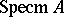, which is the subspace ofconsisting of the closed points. For a graded ringone also considers the projective spectrum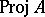. If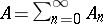, then the points ofare the homogeneous prime idealsofsuch that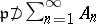.